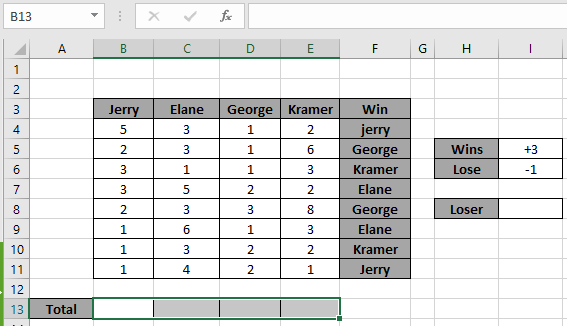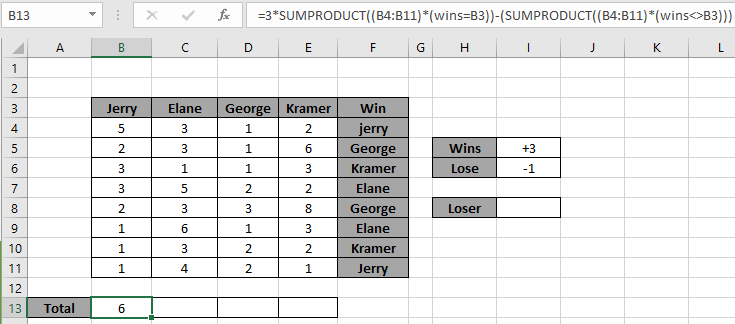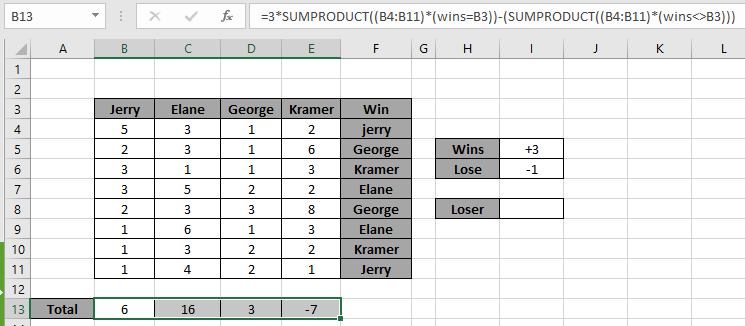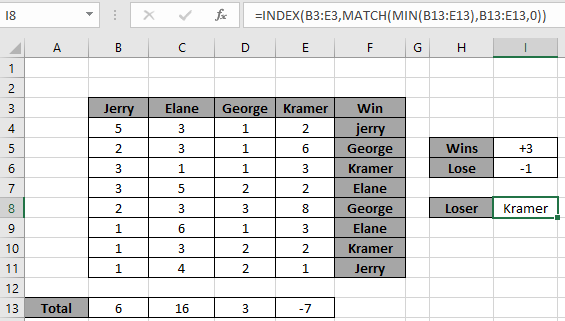# Find the Loser in game with criteria in Excel

In this article, we will learn how to find the Loser in a game of points with criteria in Excel.

Scenario :

Working with game data, sometimes we need to get points table for each team playing. Points are based on the logic that if team wins it will get plus points and if losses pints are deducted. Let's understand how to get the correct points table using the Excel formula explained below.

How to solve the problem ?

For this article we will be required to use the SUMPRODUCT function. Now we will make a formula out of the mentioned functions. Here we are given a list of points and a list of winners for the set. We need to find the loser. We will calculate the points gained and points lost in the same formula

Generic formula for correct responses:

= win_point * SUMPRODUCT ( ( points ) * ( wins = P1 ) ) - lose_point ( SUMPRODUCT ( ( points ) * ( wins <> P1 ) ) )

win_point : win percentage

lose_point : lose percentage

points : points by player 1

wins : winner of each game

P1 : Player 1

Example:

All of these might be confusing to understand. So, let's test this formula via running it on the example shown below.

Game :

The game dataset is shown below. Here each player mentions his target points for each game played. He or She has to reach the target in order to win the match. The points criteria is based on the given values as *3 for the win match and -1 for lost match.Here the named ranges are used to provide fixed array to the formula. Named ranges used for the array F4:F11. Firstly,  we need to find the points for player 1.

Use the Formula:

= 3 * SUMPRODUCT ( ( B4:B11 ) * ( wins = B3 ) ) - ( SUMPRODUCT ( ( B4:B11 ) * ( wins <> B3 ) ) )

Explanation:

• SUMPRODUCT matches all the points matching the win array with each player for each match.
• First formula gets the win points and the second formula gets the lost points.
• Each formula returns points and SUMPRODUCT gets the SUM.First player scores 6 points after all the games played. Now to get the score for each player Use Ctrl + R or drag right with already input formula cell.Here are all the points scored by each player. Use this formula for the larger data and leave the calculation for the formula.

Now we need to find the loser for the game. Loser can be evaluated using the INDEX and MATCH function given below.
Use the formula:

= INDEX ( B3:E3 , MATCH ( MIN ( B13:E13 ) , B13:E13 , 0 ) )Kramer lost the game and Elane won the game. That's interesting.

Here are some observational notes shown below.

Notes:

1. The formula only works with numbers.
2. The MATCH function takes 0 as an argument to get the exact match from the list.
3. The SUMPRODUCT function considers non - numeric values ( like text abc ) and error values ( like #NUM! , #NULL! )  as null values.
4. The SUMPRODUCT function considers logic value TRUE as 1 and False as 0.
5. The argument array must be of the same length else the function.

Hope this article about how to Return Sum of top 5 values or bottom 5 values with criteria in Excel is explanatory. Find more articles on SUMPRODUCT functions here. Please share your query below in the comment box. We will assist you.

If you liked our blogs, share it with your friends on Facebook. And also you can follow us on Twitter and Facebook. We would love to hear from you, do let us know how we can improve, complement or innovate our work and make it better for you. Write us at info@exceltip.com

Related Articles

How to use the SUMPRODUCT function in Excel: Returns the SUM after multiplication of values in multiple arrays in excel.

SUM if date is between : Returns the SUM of values between given dates or period in excel.

Sum if date is greater than given date: Returns the SUM of values after the given date or period in excel.

2 Ways to Sum by Month in Excel: Returns the SUM of values within a given specific month in excel.

How to Sum Multiple Columns with Condition: Returns the SUM of values across multiple columns having condition in excel

How to use wildcards in excel : Count cells matching phrases using the wildcards in excel

Popular Articles

50 Excel Shortcut to Increase Your Productivity : Get faster at your task. These 50 shortcuts will make you work even faster on Excel.

How to use the VLOOKUP Function in Excel : This is one of the most used and popular functions of excel that is used to lookup value from different ranges and sheets.

How to use the COUNTIF function in Excel : Count values with conditions using this amazing function. You don't need to filter your data to count specific values. Countif function is essential to prepare your dashboard.

How to Use SUMIF Function in Excel : This is another dashboard essential function. This helps you sum up values on specific conditions.

Terms and Conditions of use

The applications/code on this site are distributed as is and without warranties or liability. In no event shall the owner of the copyrights, or the authors of the applications/code be liable for any loss of profit, any problems or any damage resulting from the use or evaluation of the applications/code.